# CLASS 12 MATHS CHAPTER 3-MATRICES

### Solve The Following Questions.

Question1. In the matrix A =, write:

(i) The order of the matrix.

(ii) The number of elements.

(iii) Write the elements

Solution :
(i) There are 3 horizontal lines (rows) and 4 vertical lines (columns) in the given matrix A.

Therefore, Order of the matrix is 3 x 4.

(ii) The number of elements in the matrix A is 3 x 4 = 12.

(iii) a13 Element in first row and third column = 19

a21 Element in second row and first column = 35

a33 Element in third row and third column = -5

a24 Element in second row and fourth column = 12

a23 Element in second row and third column = 5/2

Question2. If a matrix has 24 elements, what are possible orders it can order? What, if it has 13 elements?

Solution :
We know that if a matrix is of the order m × n, it has mn elements. Thus, to find all the possible orders of a matrix having 24 elements, we have to find all the ordered pairs of natural numbers whose product is 24.

The ordered pairs are: (1, 24), (24, 1), (2, 12), (12, 2), (3, 8), (8, 3), (4, 6), and (6, 4)

Hence, the possible orders of a matrix having 24 elements are:

1 × 24, 24 × 1, 2 × 12, 12 × 2, 3 × 8, 8 × 3, 4 × 6, and 6 × 4

(1, 13) and (13, 1) are the ordered pairs of natural numbers whose product is 13.

Hence, the possible orders of a matrix having 13 elements are 1 × 13 and 13 × 1.

Question3. If a matrix has 18 elements, what are the possible orders it can have? What if has 5 elements?

Solution :
We know that if a matrix is of the order m × n, it has mn elements. Thus, to find all the possible orders of a matrix having 18 elements, we have to find all the ordered pairs of natural numbers whose product is 18.

The ordered pairs are: (1, 18), (18, 1), (2, 9), (9, 2), (3, 6,), and (6, 3)

Hence, the possible orders of a matrix having 18 elements are:

1 × 18, 18 × 1, 2 × 9, 9 × 2, 3 × 6, and 6 × 3

(1, 5) and (5, 1) are the ordered pairs of natural numbers whose product is 5.

Hence, the possible orders of a matrix having 5 elements are 1 × 5 and 5 × 1.

Question4. Construct a 2 x 2 matrix A = [aij ] whose elements are given by:

(i)

(ii) aij = i/j

(iii)

Solution :
(i)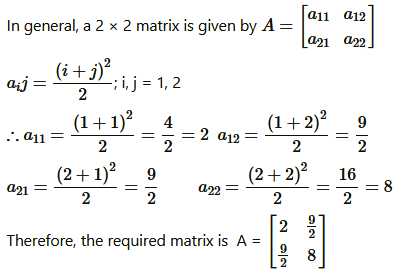(ii)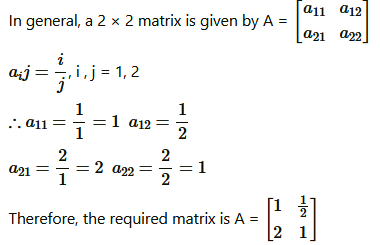(iii)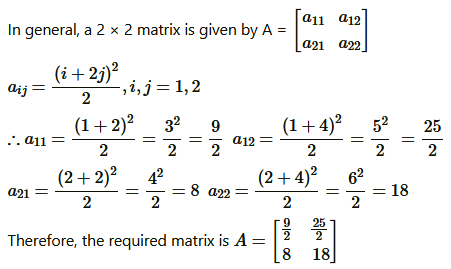Question5. Construct a 3 x 4 matrix, whose elements are given by:

(i)

(ii) aij = 2i – j

Solution :
(i)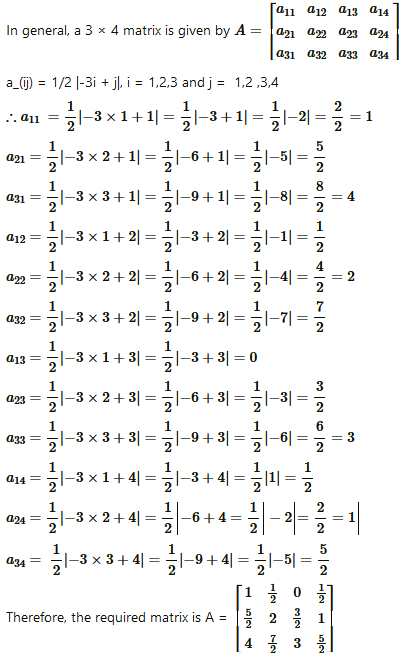(ii)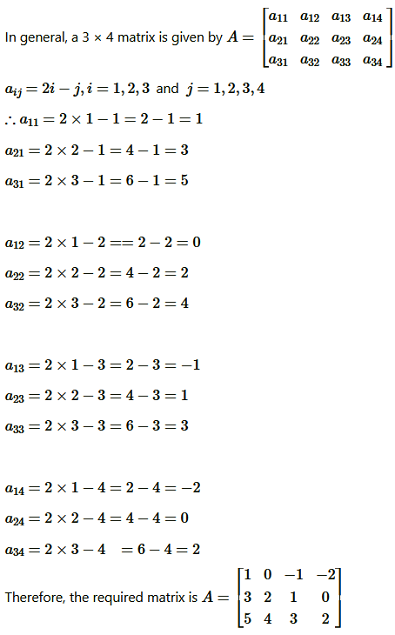Question6. Find the values of x,y and z from the following equations:

(i)

(ii)

(iii)

Solution :
(i)Given:

As the given matrices are equal, their corresponding elements are also equal.

Comparing the corresponding elements, we get:

x = 1, y = 4 = z = 3

(ii)

As the given matrices are equal, their corresponding elements are also equal.

Comparing the corresponding elements, we get:

x + y = 6, xy = 8 and 5 + z = 5

Now, 5 + z = 5 ⇒ z = 0

We know that:

(x – y)2 = (x + y)2 – 4xy

⇒  (x – y)2 = 36 – 32 = 4

⇒x – y = ± 2

Now, when x – y = 2 and x + y = 6  , we get  x = 4, y = 2

When x – y = -2 and x + y = 6, we get  x = 2, y = 4

∴ x  = 4, y = 2, and z = 0,  or x = 2, y = 4 and z = 0

(iii)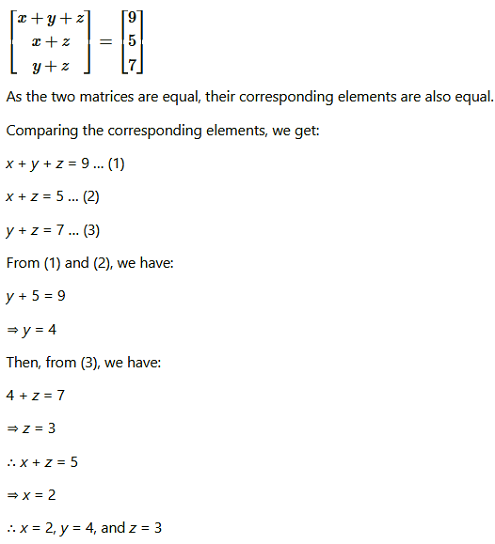Question7. Find the values of a,b, c and d from the equation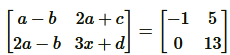.

Solution :As the two matrices are equal, their corresponding elements are also equal.

Comparing the corresponding elements, we get:

a − b = −1 … (1)

2a − b = 0 … (2)

2a + c = 5 … (3)

3c + d = 13 … (4)

From (2), we have:

b = 2a

Then, from (1), we have:

a − 2a = −1

⇒ a = 1

⇒ b = 2

Now, from (3), we have:

2 ×1 + c = 5

⇒ c = 3

From (4) we have:

3 ×3 + d = 13

⇒ 9 + d = 13 ⇒ d = 4

∴a = 1, b = 2, c = 3, and d = 4

Question 8. A = [aij]m x n is a square matrix if:

(A) m < n (B) m > n  (C) m = n (D) None of these

Solution :
It is known that a given matrix is said to be a square matrix if the number of rows is equal to the number of columns. A = [aij]m x n

option (C) is correct.

Question9. Which of the given values of  x and y make the following pairs of matrices equal:

(B) Not possible to find

Solution :

We find that on comparing the corresponding elements of the two matrices, we get two different values of x , which is not possible.

Hence, it is not possible to find the values of  x and y  for which the given matrices are equal.

Therefore, option (B) is correct.

Question10. The number of all possible matrices of order 3 x 3 with each entry 0 or 1 is:

(A) 27

(B) 18

(C) 81

(D) 512

Solution :

The given matrix of the order 3 × 3 has 9 elements and each of these elements can be either 0 or 1.

Now, each of the 9 elements can be filled in two possible ways.

Therefore, by the multiplication principle, the required number of possible matrices is 29 = 512

### Solve The Following Questions.

Question1. Let A = . Find each of the following:

(i) A + B

(ii) A – B

(iii) 3A – C

(iv) AB

(v) BA

Solution :
(i)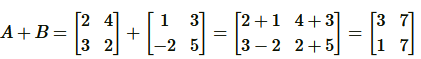(ii)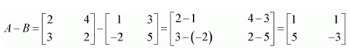(iii)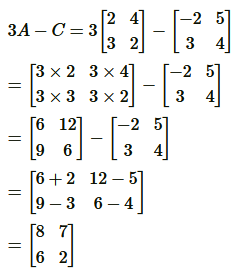(iv)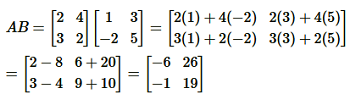(v)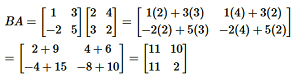Question2. Compute the following:

(i)

(ii)

(iii)

(iv)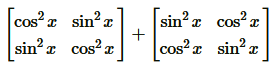Solution :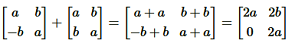(ii)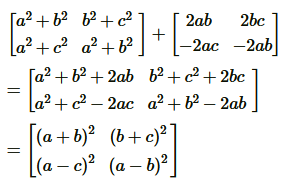(iii)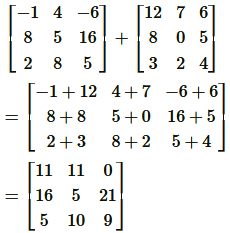(iv)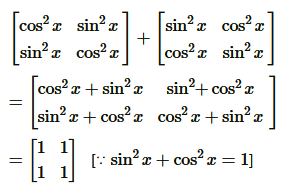Question3. Compute the indicated products:

(i)

(ii)

(iii)

(iv)

(v)

(vi)

Solution :
(i)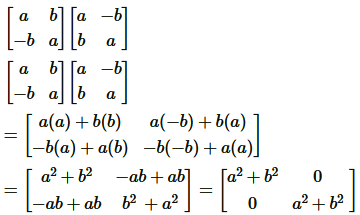(ii)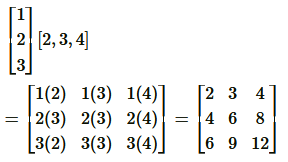(iii)  =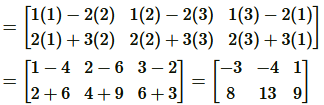(iv)=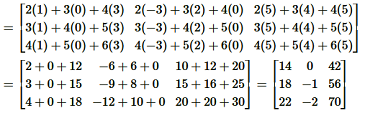(v)=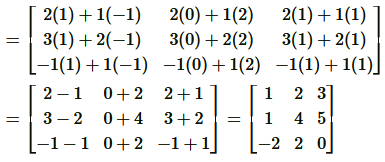(vi)=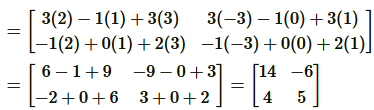Question4. If    then compute (A + B) and (B – C). Also, verify that A + (B – C) = (A + B) – C.

Solution :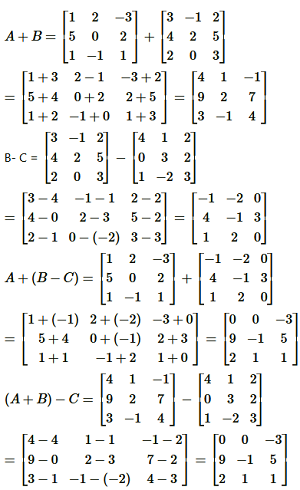L.H.S. = R.H.S.  Proved.

Question5. Ifthen compute 3A – 5B.

Solution :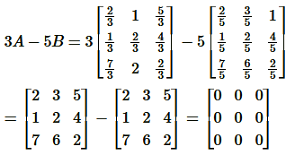Question6. Simplify:

Solution :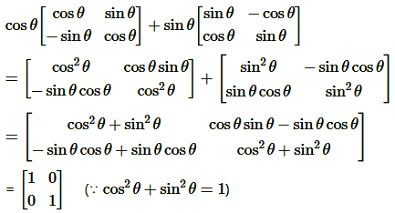Question7. Find X and Y, if:

(i)

(ii)

Solution :
(i)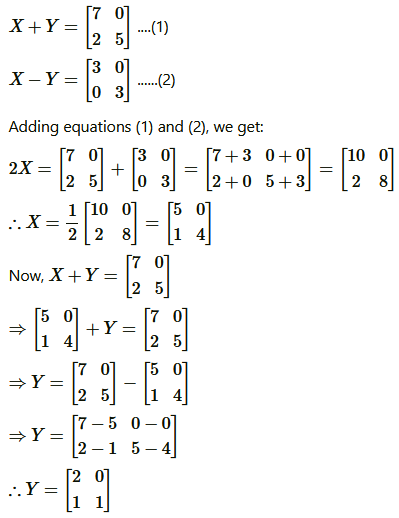(ii)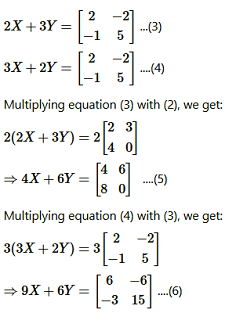Question8. Find X if Y =

Solution :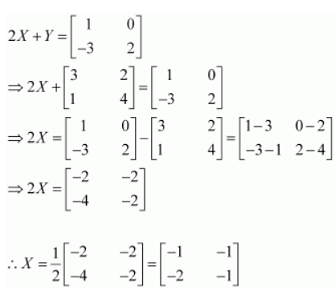Question9. Find x and y if

Solution :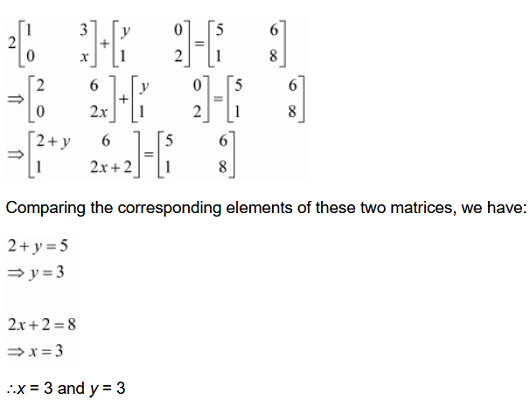Question10. Solve the equation for x,y,z and t if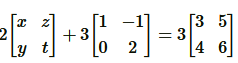Solution :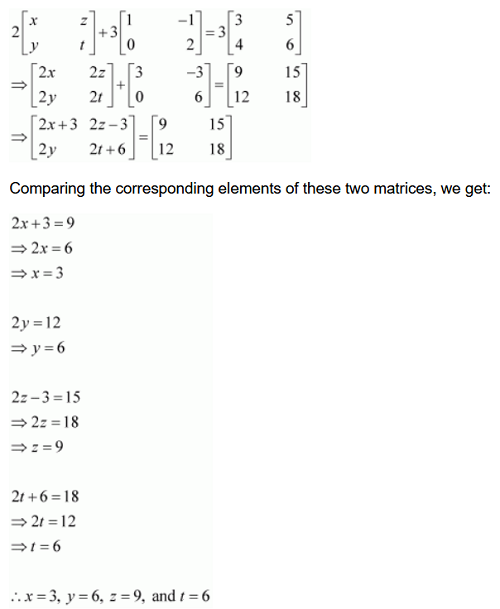Question11. Iffind the values of x and y

Solution :

Comparing the corresponding elements of these two matrices, we get:

2x − y = 10 and 3x + y = 5

Adding these two equations, we have:

5x = 15

⇒ x = 3

Now, 3x + y = 5

⇒ y = 5 − 3x

⇒ y = 5 − 9 = −4

∴x = 3 and y = −4

Question12. Given: find the values of x ,y,z and w

Solution :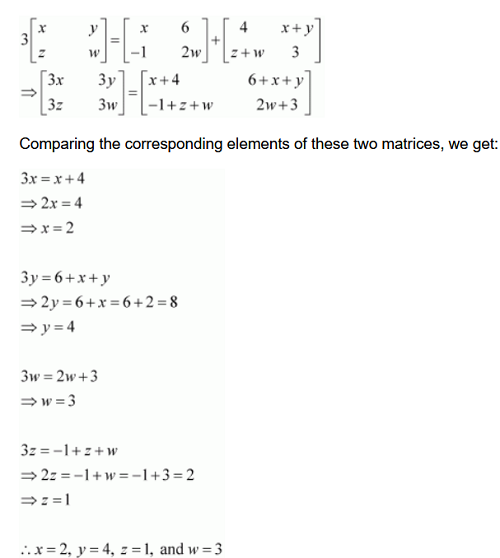Question13. If show that F(x) F(y) = F(x + y)

Solution :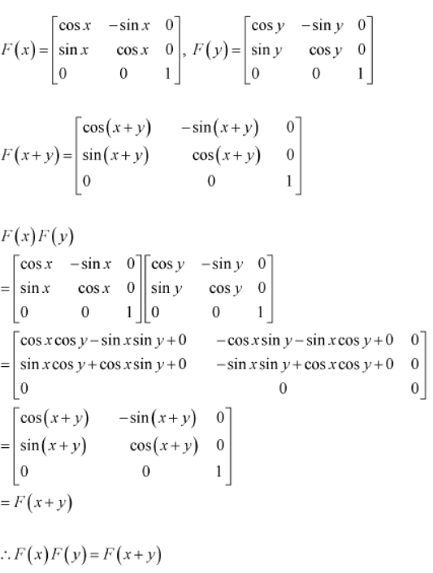Question14. Show that:

(i)

(ii)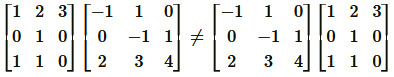Solution :
(i)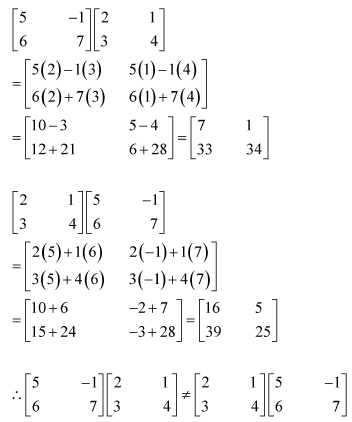(ii)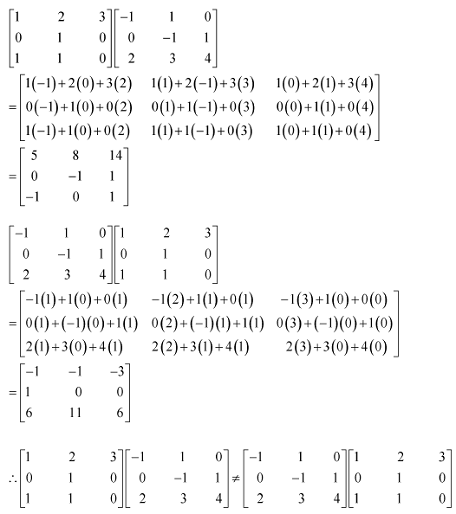Question15. Find A– 5A + 6I if A = .

Solution: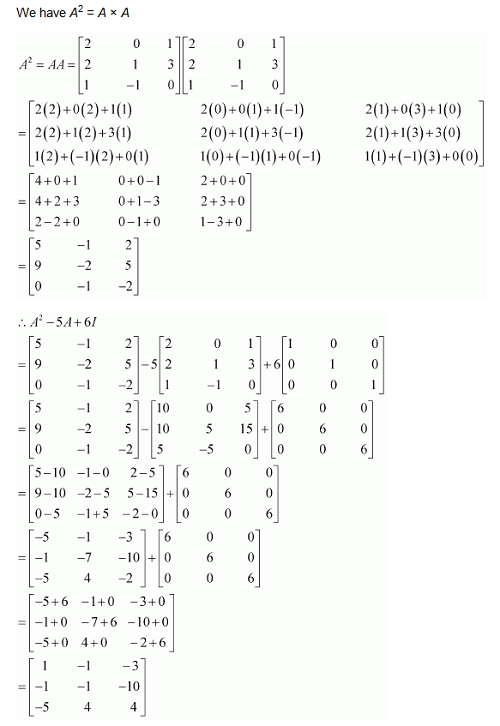Question16. If A =  prove that A3 – 6A+ 7A + 2I = 0.

Solution :
L.H.S. = A3 – 6A2 + 7A + 2I

= R.H.S.      Proved.

Question17. If   find k so that A2 = kA – 2I

Solution :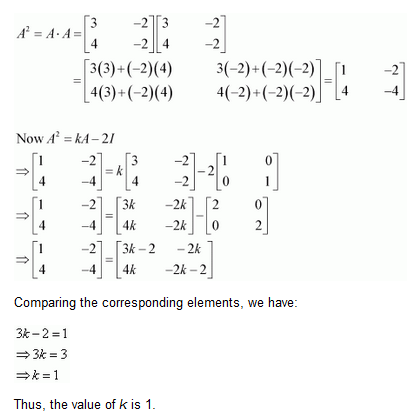Question18. If   and I is the identity matrix of order 2, show that I + A = (I  – A)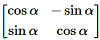Solution :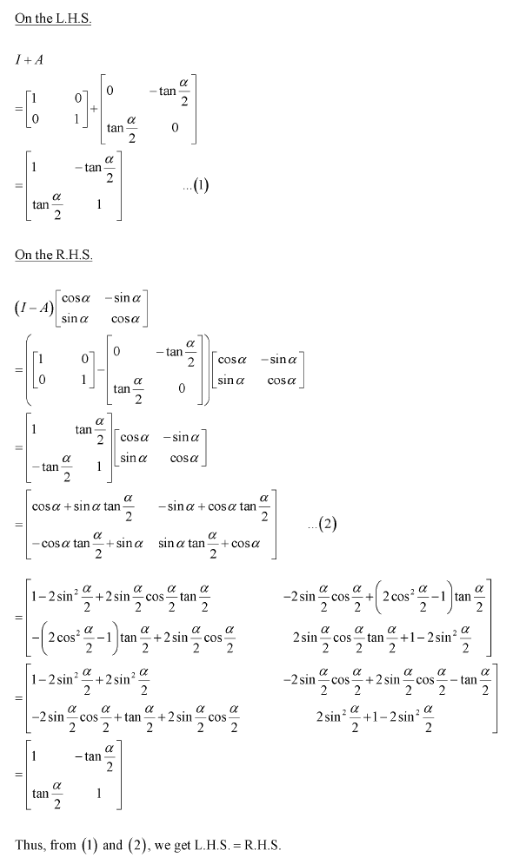Question19. A trust fund has ` 30,000 that must be invested in two different types of bond. The first bond pays 5% interest per year and the second bond pays 7% interest per year. Using matrix multiplication, determine how to divide ` 30,000 in two types of bonds, if the trust fund must obtain an annual interest of (a) ` 1800, (b) ` 2000.

Solution :
(a) It is given that Rs.30,000 must be invested into two types of bonds with 5% and 7% interest rates.

Let Rs. x be invested in bonds of the first type. Thus, Rs. (30,000 − x) will be invested in the other type.

Hence, the amount invested in each type of the bonds can be represented in matrix form with each column corresponding to a different type of bond as:

X = [x ​30,000 − x​]

Annual interest obtained is Rs. 1800. We know, Interest = PTR/100

Here, the time is one year and thus T = 1.

Hence, the interest obtained after one year can be expressed in matrix representation as-

⇒  5x + 210000 − 7x = 180000

⇒ – 2x = – 30000

∴  x = 15000

Amount invested in the first bond = x = Rs. 15000

⇒ Amount invested second bond = Rs. (30000 − x) = Rs. (30000 − 15000) = Rs. 15000

∴ The trust has to invest Rs. 15000 each in both the bonds in order to obtain an annual interest of Rs. 1800.

(b) It is given that Rs.30,000 must be invested into two types of bonds with 5% and 7% interest rates.

Let Rs. x be invested in bonds of the first type. Thus, Rs. (30,000 − x) will be invested in the other type.

Hence, the amount invested in each type of the bonds can be represented in matrix form with each column corresponding to a different type of bond as:

X = [x ​30,000 − x​]

Annual interest obtained is Rs. 2000.

Hence, the interest obtained after one year can be expressed in matrix representation as-

⇒  5x + 210000 − 7x = 200000

⇒  −2x = − 10000

∴  x = 5000

Amount invested in the first bond = x = Rs. 5000

⇒ Amount invested second bond = Rs. (30000 − x) = Rs. (30000 − 5000) = Rs. 25000

∴ The trust has to invest Rs.5000 in the first bond and Rs.25000 in the second bond in order to obtain an annual interest of Rs.2000.

Question20. The bookshop of a particular school has 10 dozen chemistry books, 8 dozen physics books, 10 dozen economics books. Their selling prices are ` 80, ` 60 and ` 40 each respectively. Find the total amount the bookshop will receive from selling all the books using matrix algebra.

Solution :
The bookshop has 10 dozen chemistry books, 8 dozen physics books, and 10 dozen economics books.

The selling prices of a chemistry book, a physics book, and an economics book are respectively given as Rs 80, Rs 60, and Rs 40.

The total amount of money that will be received from the sale of all these books can be represented in the form of a matrix as:

Thus, the bookshop will receive Rs 20160 from the sale of all these books.

Question21. Assume X, Y, Z, W and P are matrices of order 2 x n,3 x k,2 x p, n x 3 and p x k repectively. The restriction on n,k and p so that PY + WY will be define are:

A. k = 3, p = n

B. k is arbitrary, p = 2

C. p is arbitrary, k = 3

D. k = 2, p = 3

Solution :
Matrices P and Y are of the orders p × k and 3 × k respectively.

Therefore, matrix PY will be defined if k = 3. Consequently, PY will be of the order p × k.

Matrices W and Y are of the orders n × 3 and 3 × k respectively.

Since the number of columns in W is equal to the number of rows in Y, matrix WY is well-defined and is of the order n × k.

Matrices PY and WY can be added only when their orders are the same.

However, PY is of the order p × k and WY is of the order n × k. Therefore, we must have p = n.

Thus, k = 3 and p = n are the restrictions on n, k, and p so that PY + WY will be defined.

Therefore, option (A) is correct.

Question22. Assume X, Y, Z, W and P are matrices of order 2 x n,3 x k,2 x p, n x 3 and p x k repectively. If n = p then order of matrix 7X – 5Z is:

(A) p × 2

(B) 2 × n

(C) n × 3

(D)p × n

Solution :

Matrix X is of the order 2 × n.

Therefore, matrix 7X is also of the same order.

Matrix Z is of the order 2 × p, i.e., 2 × n    [Since n = p]

Therefore, matrix 5Z is also of the same order.

Now, both the matrices 7X and 5Z are of the order 2 × n.

Thus, matrix 7X − 5Z is well-defined and is of the order 2 × n.

### Solve The Following Questions.

Question1. Find the transpose of each of the following matrices:

(i)

(ii)

(iii)

Solution :
(i) Let A =

Transpose of A = A’ or AT = [ 5    1/2     -1]

(ii)

Transpose of A = A’ or AT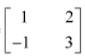(iii)

Transpose of A = A’ or AT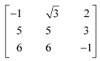Question2. If   then verify that:

(i) (A + B)’ = A’ + B’

(ii) (A – B)’ = A’ – B’

Solution :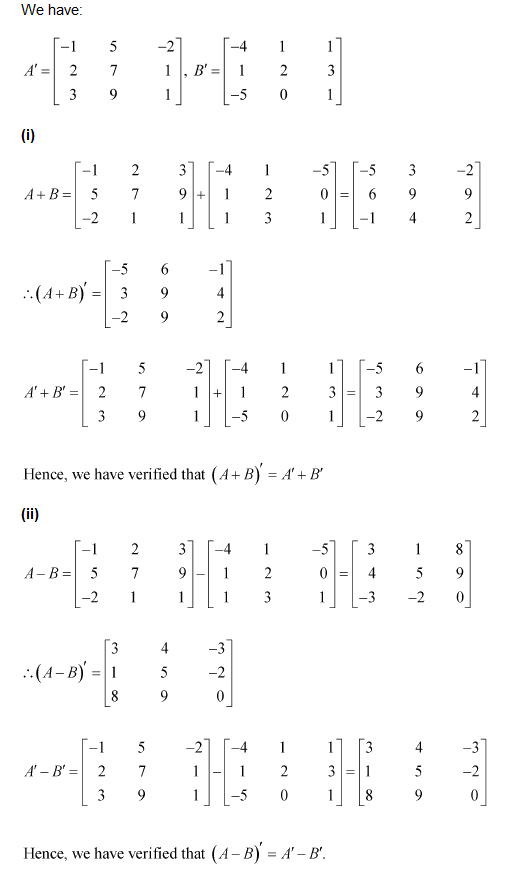Question3. If   then verify that:

(i) (A + B)’ = A’ + B’

(ii)  (A – B)’ = A’ – B’

Solution :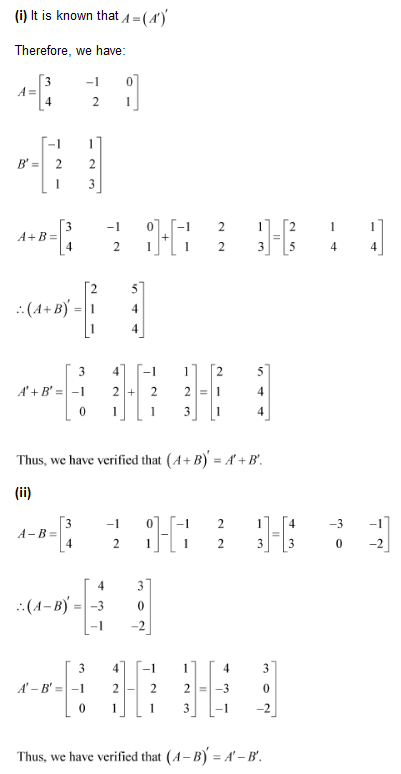Question4. If   then find (A + 2B)’.

Solution :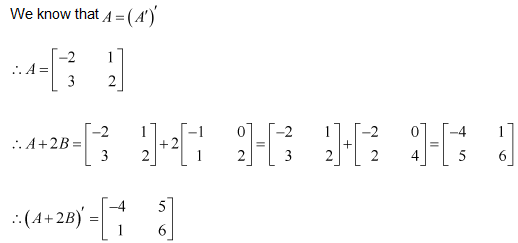Question5. For the matrices A and B, verify that (AB)’ = B’A’, where:

(i)

(ii)

Solution :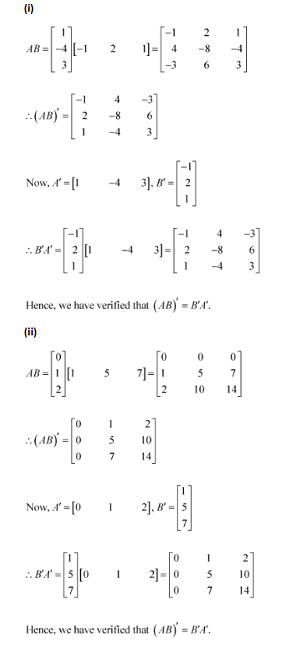Question6. (i) If A =  then verify that A’A = I.

(ii) If A =  then verify that A’A = I.

Solution :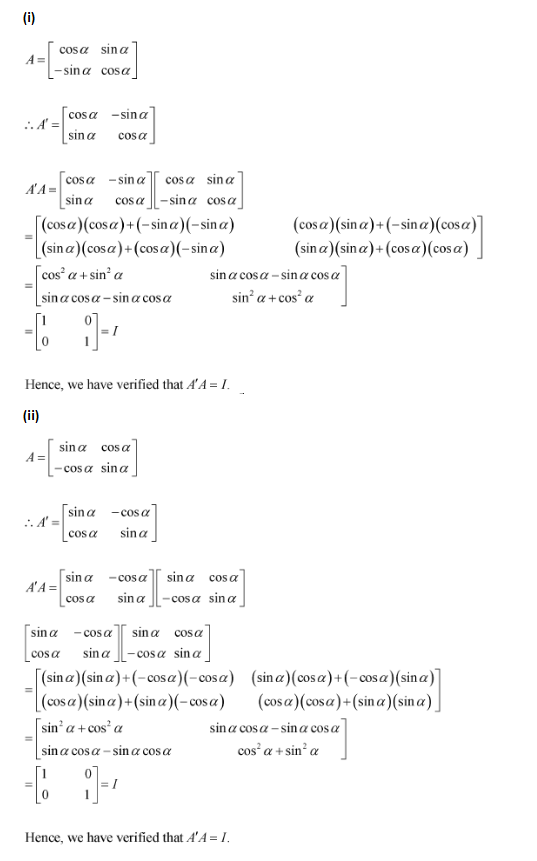Question7. (i) Show that the matrix A =  is a symmetric matrix.

(ii) Show that the matrix A =  is a skew symmetric matrix.

Solution :
(i) Given: A =

Changing rows of matrix A as the columns of new matrix A’ =  = A

∴ A’ = A

Therefore, by definitions of symmetric matrix, A is a symmetric matrix.

(ii) Given: A =

A’ =  = – = – A

∴ A’ = – A

Therefore, by definition matrix A is a skew-symmetric matrix

Question8. For a matrix A =  verify that:

(i) (A + A’) is a symmetric matrix.

(ii) (A – A’) is a skew symmetric matrix.

Solution :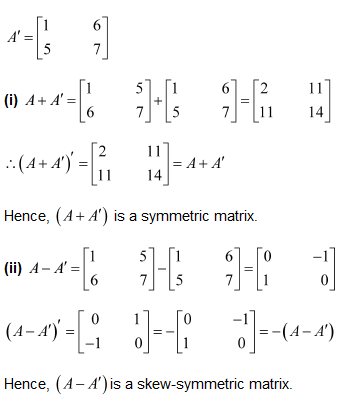Question9. Find 1/2 (A + A’) and 1/2(A – A’) when A =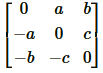Solution :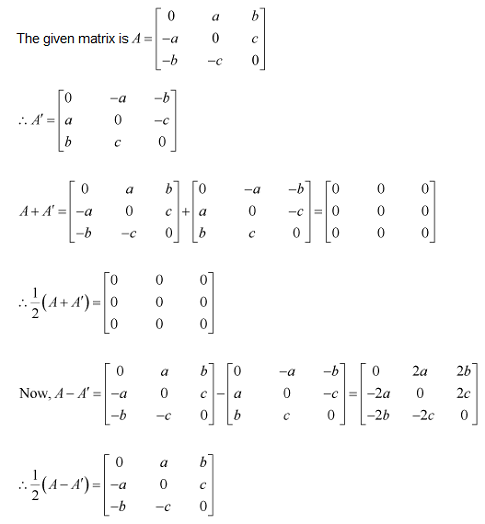Question10. Express the following matrices as the sum of a symmetric and skew symmetric matrix:

(i)

(ii)

(iii)

(iv)

Solution :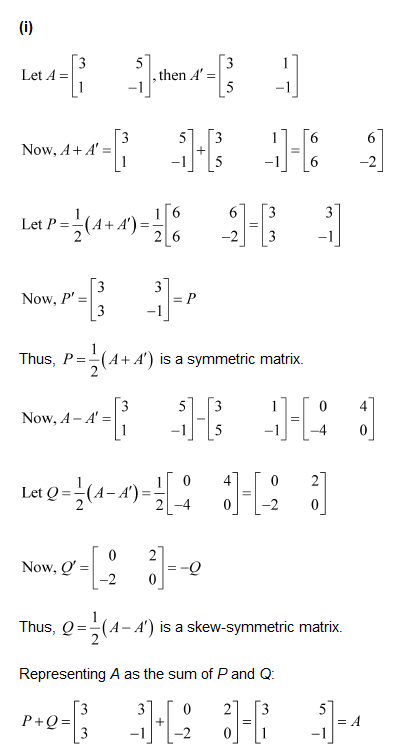(iii)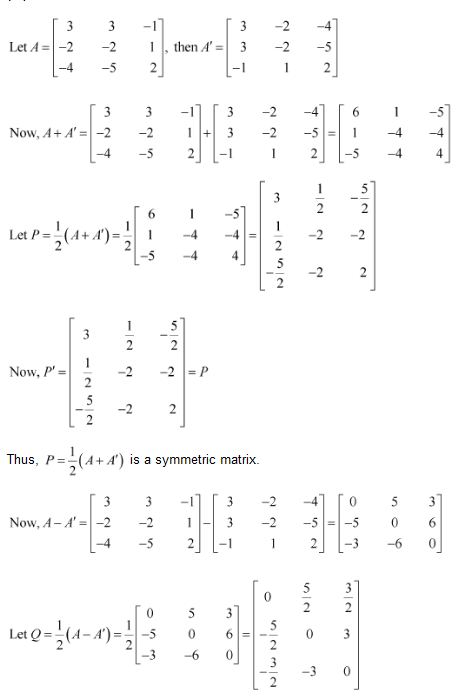(iv)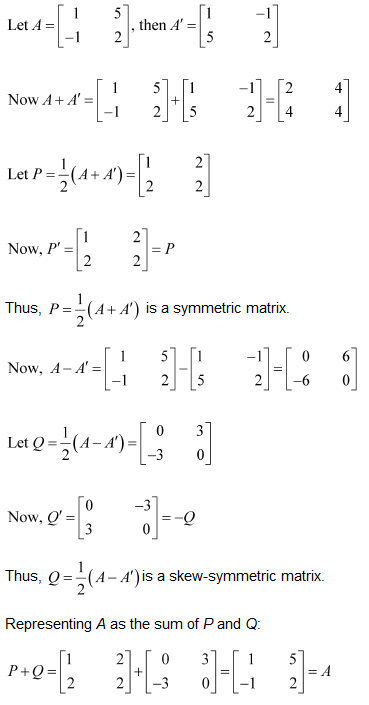Choose the correct answer in Exercises 11 and 12.

Question11. If A and B are symmetric matrices of same order, AB – BA is a:

(A) Skew-symmetric matrix

(B) Symmetric matrix

(C) Zero matrix

(D) Identity matrix

Solution :
Given: A and B are symmetric matrices ∴ A = A’ and B = B’

Now, (AB – BA)’ = (AB)’ – (BA)’  ∴ (AB – BA)’ = B’A’ – A’B’ [Reversal law]

∴ (AB – BA)’ = BA – AB [From eq. (i)] ∴ (AB – BA)’ = – (AB – BA)

∴  (AB – BA) is a skew matrix.

Therefore, option (A) is correct.

Question12. If A = , then A + A’ = I, if the value of α is:

(A) π/6

(B) π/3

(C) π

(D) 3π/2

Solution :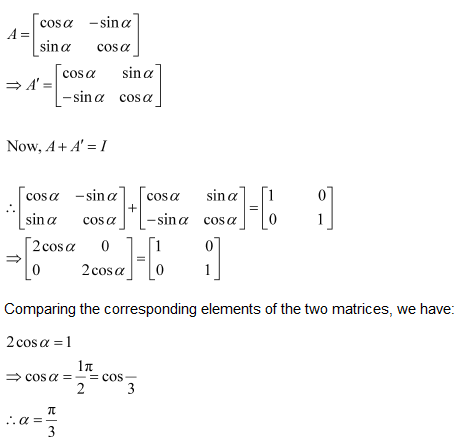Therefore, option (B) is correct.

### Solve The Following Questions.

Using elementary transformation, find the inverse of each of the matrices, if it exists in Exercises 1 to 6.

Question1.

Solution :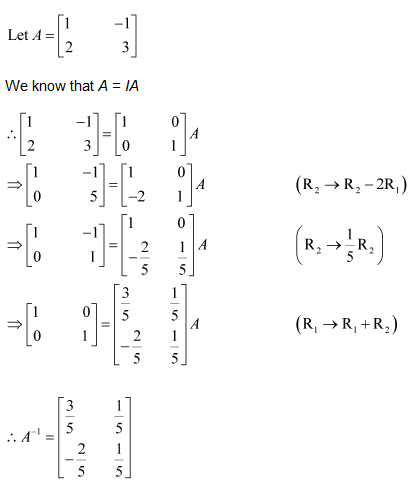Question2.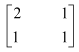Solution :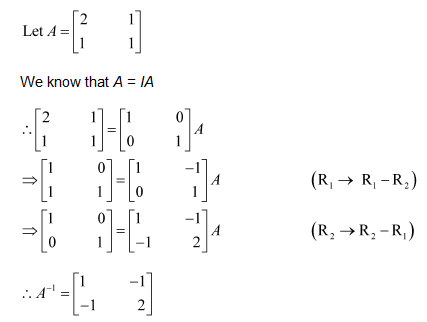Question3.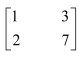Solution :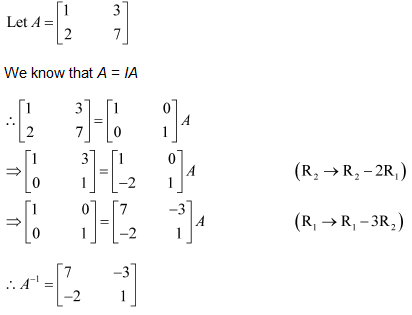Question4.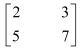Solution :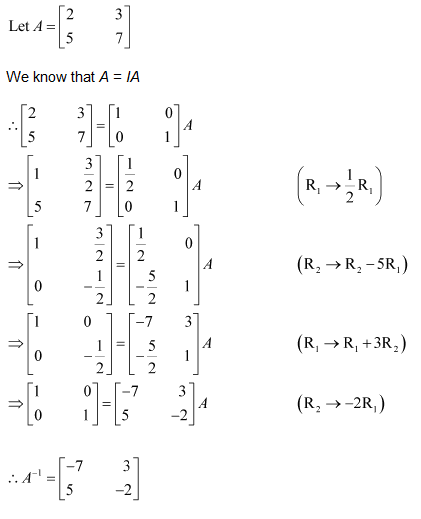Question5.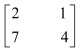Solution :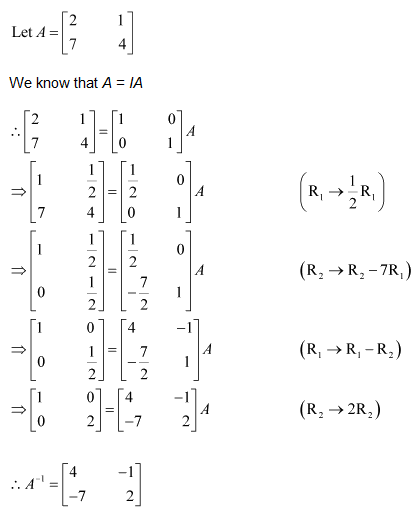Question6.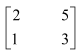Solution :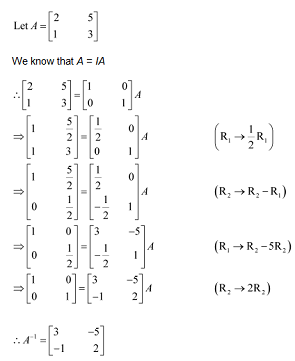Using elementary transformation, find the inverse of each of the matrices, if it exists in Exercises 7 to 14.

Question7.

Solution :

Question8.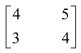Solution :
Let A =Question9.

Solution :
Let A =

Question10.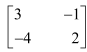Solution :
Let A =Question11.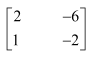Solution :
Let A =Question12.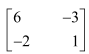Solution :
Let A =Question13.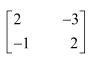Solution :
Let A =Question14.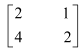Solution :
Let A =Using elementary transformation, find the inverse of each of the matrices, if it exists in Exercises 15 to 17.

Question15.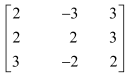Solution :
Let A =Question16.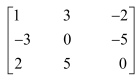Solution :
Let A =,

Question17.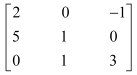Solution :
Let A =,

Question18.  Matrices A and B will be inverse of each other only if:

(A) AB = BA

(B) AB = BA = 0

(C) AB = 0, BA = I

(D) AB = BA = I

Solution :
By definition of inverse of square matrix,

Option (A) is correct.

### Solve The Following Questions.

Question1. Let A =  show that  where I is the identity matrix of order 2 and ∈ N.

Solution :

Given:

Question2. If A = , prove that An=  ,n ∈ N.

Solution :
Given: A =

To show :P(n): An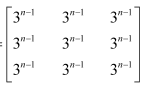,n ∈ N

We shall prove the result by using the principle of mathematical induction.

For n = 1, we have:

Question3. If A =  then prove that An =  where n is any positive integer.

Solution :
Given: An =

To show :P(n): An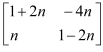,n ∈ N

We shall prove the result by using the principle of mathematical induction.

For n = 1, we have:

Question4. If A and B are symmetric matrices, prove that AB – BA is a skew symmetric matrix.

Solution :
A and B are symmetric matrices.

A’ = A and B’ = B  ……….(i)

Now, (AB – BA)’ = (AB)’ – (BA)’          ∴ (AB – BA)’ = B’A’ – A’B’ [Reversal law]

∴ (AB – BA)’ = BA – AB   [Using eq. (i)]

∴ (AB – BA)’ = – (AB – BA)

Therefore, (AB – BA) is a skew symmetric.

Question5. Show that the matrix B’AB is symmetric or skew symmetric according as A is symmetric or skew symmetric.

Solution :
(B’AB)’ = [B’(AB]’ = (AB)’ (B’)’   [∴ (CD)’ = D’C’]

∴  (B’AB)’ = B’A’B   ……….(i)

Case I: A is a symmetric matrix, then   A’ = A

From eq. (i)  (B’AB)’ = B’AB

B’AB is a symmetric matrix.

Case II: A is a skew symmetric matrix. A’ = – A

Putting A’ = – A in eq. (i),  (B’AB)’ = B’(– A)B = – B’AB

B’AB is a skew symmetric matrix.

Question6. Find the values of x,y,z if the matrix   satisfies the equation A’A = I.

Solution :
Given:

Question7. For what value of x  = O?

Solution :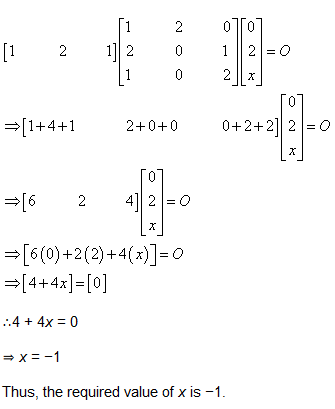Question8. If A =  show that A– 5A + 7I = 0.

Solution :
Given: A =

Question9. Find x  if

Solution :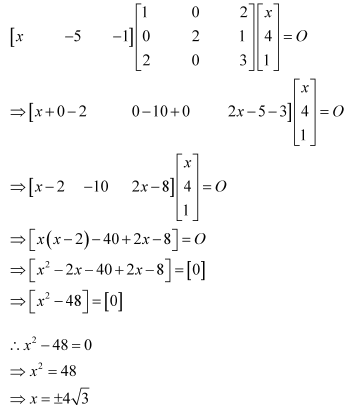Question10. A manufacturer produces three products, x,y,z  which he sells in two markets. Annual sales are indicated below:

(a) If unit sales prices of x,y and z are ` 2.50, ` 1.50 and ` 1.00 respectively, find the total revenue in each market with the help of matrix algebra.

(b) If the unit costs of the above three commodities are ` 2.00, ` 1.00 and 50 paise respectively. Find the gross profit.

Solution :

(a) The unit sale prices of x, y, and z are respectively given as Rs 2.50, Rs 1.50, and Rs 1.00.

Consequently, the total revenue in market I can be represented in the form of a matrix as:

= 10000 x 2.50 + 2000 x 1.50 + 18000 x 1.00

= 25000 + 3000 + 18000

= 46000

The total revenue in market II can be represented in the form of a matrix as:

= 6000 x 2.50 + 20000 x 1.50 + 8000 x 1.00

= 15000 + 30000 + 8000

= 53000

Therefore, the total revenue in market I isRs 46000 and the same in market II isRs 53000.

(b) The unit cost prices of x, y, and z are respectively given as Rs 2.00, Rs 1.00, and 50 paise.

Consequently, the total cost prices of all the products in market I can be represented in the form of a matrix as: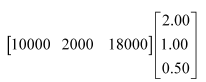= 10000 x 2.00 + 2000 x 1.00 + 18000 x 0.50

= 20000 + 2000 + 9000

= 31000

Since the total revenue in market I isRs 46000, the gross profit in this marketis (Rs 46000 − Rs 31000) Rs 15000.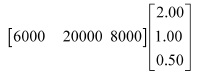= 6000 x 2.00 + 20000 x 1.00 + 8000 x 0.50

= 12000 + 20000 + 4000

= 36000
The total cost prices of all the products in market II can be represented in the form of a matrix as:

Since the total revenue in market II isRs 53000, the gross profit in this market is (Rs 53000 − Rs 36000) Rs 17000.

Question11. Find the matrix X so that X

Solution :
Given: X  ……….(i)

The matrix given on the R.H.S. of the equation is a 2 × 3 matrix and the one given on the L.H.S. of the equation is a 2 × 3 matrix. Therefore, X has to be a 2 × 2 matrix.

Question12. If A and B are square matrices of the same order such that AB = BA, then prove by induction that AB’’ = B’’A. Further prove that (AB)’’ = A’’B’’ for all n ∈ N.

Solution :
Given: AB = BA …..(i)

Now, we prove that the result is true for n = k + 1.

Therefore, the result is true for n = k + 1.

Thus, by the principle of mathematical induction, we have (AB)’’ = A’’B’’, for all natural numbers.

Question13. If A =  is such that A2 = I, then:

Solution :
Given:  and A2 = I

Equating corresponding entries, we haveTherefore, option (C) is correct.

Question14. If the matrix A is both symmetric and skew symmetric, then:

(A) A is a diagonal matrix

(B) A is a zero matrix

(C) A is a square matrix

(D) None of these

Solution :
Since, A is symmetric, therefore, A’ = A ……..(i)

And A is skew-symmetric, therefore, A’ = – A

A = – A  [From eq. (i)]

A + A = 0 ⇒2A = 0  ⇒A = 0

Therefore, A is zero matrix.

Therefore, option (B) is correct.

Question15. If A is a square matrix such that A2 = A, then (I + A)3 – 7A is equal to:

(A) A

(B) I – A

(C) I

(D) 3A

Solution :
Given: A2 = A    …..(i)

Multiplying both sides by A,  A3 = A2 = A [From eq. (i)]  ……(ii)

Also given (I + A)3 – 7A = I3 + A3 + 3I2A + 3IA2 – 7A

Putting A2 = A [from eq. (i)] and A3 = A [from eq. (ii)],

= I + A + 3IA + 3IA – 7A = I + A + 3A + 3A – 7A   [ IA = A]

= I + 7A – 7A = I

Therefore, option (C) is correct.

Get 30% off your first purchase!

X
error: Content is protected !!
Scroll to Top